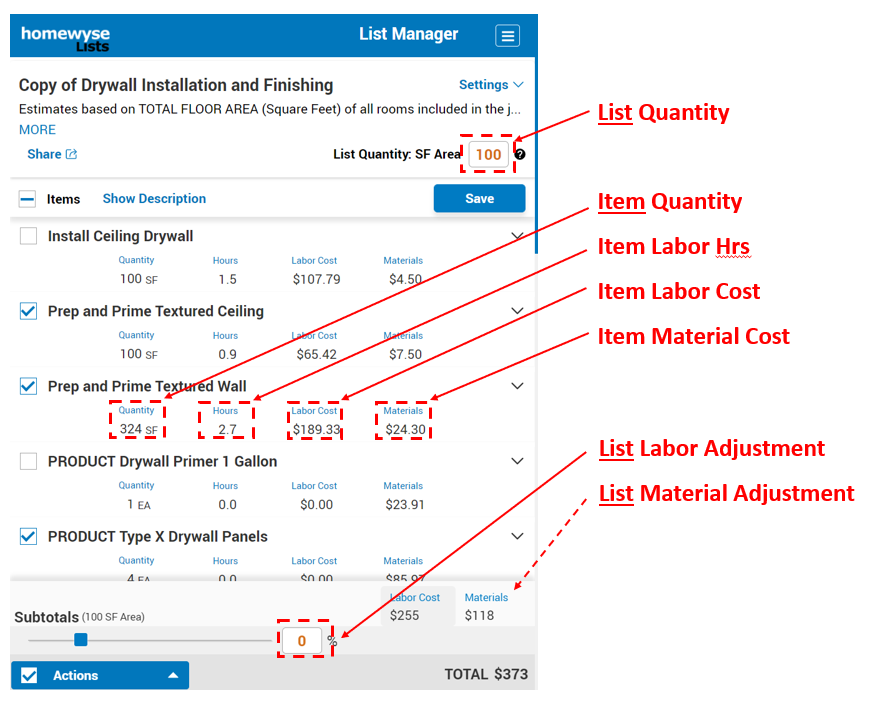# Homewyse Lists

Cost Estimation Calculations

New to Lists? Review the Getting Started Tutorial and the Lists User Guide.

The "Item" is basic element of work and cost in Homewyse Lists. Customizing a List amounts to changing or customizing the Items in that List. The following explains Item calculations and how to customize these calculations.

## Item values explained

Each Item has 4 values - these are displayed in every List from left to right:• Item Quantity This is the amount or size of each Item. The Item quantity is used to calculate all other Item values and is displayed next to the Item name. Each Item in a Homewyse list has its OWN quantity. Each Item quantity can be: calculated from the List Quantity, - or - manually entered by the list owner.
• Hours Total number of labor hours required to complete the Item Quantity of work. Note: if a team of 2 or more works on the Item, "Hrs" is the total number of person-hours that must be paid for, not the elapsed time that the team worked.
• Labor Cost Total labor costs for the Item.
• Material Cost Total material costs for the Item.

The following sections explain how each of these values is calculated and modified. NOTE: each value will depend on other values, so the sequence that you change values is IMPORTANT. In all cases, the Item Quantity should be changed first, followed by Item Labor and then lastly Item Labor Cost and Item Material Cost.

## Step1 calculation: Item Quantity

For any new or modified Item, you must first make sure the Item Quantity is being calculated to your satisfaction. The Item quantity can be set to a specific (fixed) value, or set to automatically vary as a specified multiple of the List quantity.

To specify a Fixed Item Quantity:

1. Select the Item Quantity.
2. Enter the Item Quantity directly.
3. Set the "Recalculate Automatically based on List Quantity" switch to the off (leftmost) position.
4. Choose "Save".

To specify that the Item Quantity vary in proportion to the List Quantity :

1. Select the Item Quantity.
2. Set the "Recalculate Automatically based on List Quantity" switch to the on (rightmost) position.
3. Either: (a) enter the Item Quantity directly OR (b) enter the "Item-to-List Quantity Ratio. Note that once one of these values has been entered, the other value will update so that Item Quantity = List Quantity * Item-to-List Quantity Ratio.
4. Choose "Save".

## Step 2 calculation: Item Hours

The number of Item Hours is calculated by: dividing the Item Quantity from Step 1 by the Quantity Produced per Hour factor:

Hours = Item Quantity / Quantity Produced per Hour

You can enter Item Hours directly, or specify the Quantity Produced per Hour and have Homewyse List calculate the Item Hours for you.

To specify Item Hours directly:

1. Select the Item Hours.
2. Enter the Item Hours directly.
3. Choose "Save".

To have Item Hours calculated from a Quantity Produced per Hour factor that you provide:

1. Select the Item Hours.
2. Enter the Quantity Produced per Hour factor.
3. Choose "Save".

## Step 3 calculation: Item Labor Cost

Labor cost for an item is calculated by: multiplying Item Hours (calculated in Step 2 above) by the Labor Wage and by the List Labor Adjustment

Labor Cost = Hours * Hourly Labor Rate * (100 + List Labor Adjustment)/100

You can enter Item Labor Cost directly, or specify the Hourly Labor Rate for the Item and have Homewyse List calculate the Labor Cost.

To specify Labor Cost directly (and have Homewyse List determine the corresponding Hourly Labor Rate):

1. Select the Item Labor Cost.
2. Enter the Item Labor Cost directly.
3. Choose "Save".

To have Item Labor Costs calculated from an Hourly Labor Rate that you provide:

1. Select the Item Labor Cost.
2. Enter your Hourly Labor Rate.
3. Choose "Save".

NOTE: By default the List Labor Adjustment is 0 - and should be set at 0 when editing Labor costs for any individual Item. Labor Adjustment affects ALL Items in the List and should be changed from 0 with CAUTION (only when you want to apply a markup or discount to ALL Labor costs). Change List Labor Adjustment in the List Subtotals section located near the bottom of the scree.

## Step 4 calculation:Item Material Cost

Material cost for an item is calculated by: multiplying the Item Quantity (calculated in Step 1 above) by the Unit Cost and by the List Material Adjustment

Material Cost = Item Quantity * Unit Cost * (100 + List Material Adjustment)/100

You can enter Item Material Cost directly, or specify the Unit Cost for the Item and have Homewyse List calculate the Material Cost.

To specify Material Cost directly (and have Homewyse List determine the corresponding Unit Cost):

1. Select the Item Material Cost.
2. Enter the Item Material Cost directly.
3. Choose "Save".

To have Item Material Costs calculated from a Unit Cost that you provide:

1. Select the Item Material Cost.